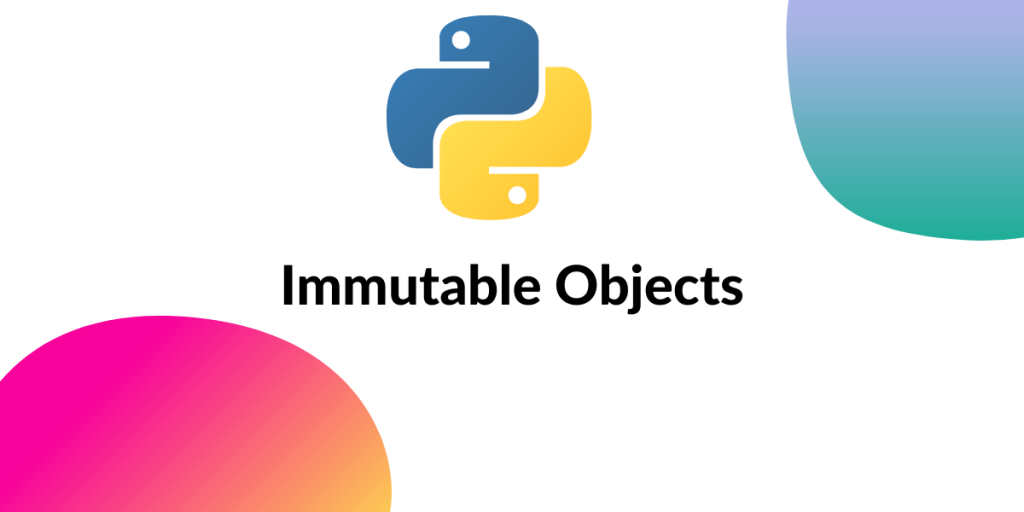# What does “Immutable” mean in Python?What does immutable mean in Python where every entity is an object? Unlike some other programming languages, where you need to explicitly specify the type of data you’re assigning to a variable, Python doesn’t require that. Instead, it automatically assigns the data type depending on the value you provide.

In short, each variable holds an object instance and is given a unique object ID which is created at the runtime of the program. The Object ID is an integer representing the memory location where the value of the variable is stored.

To get the ID of each object, you need to open Python Shell and call the default id() function and pass the variable name. Here’s an example:

```#Initializing the variable
a = "this is not a random string"

#We call the id() function with the variable name as argument
print("The Object id of 'a' is: " + str(id(a)))
```

Output:

The following output represents the

```The Object id of 'a' is: 1695893310240
```

## What is Immutability?

To understand the concept of Immutability properly, we need to know the difference between a mutable object and an immutable object.

### What is a mutable object?

If the state of an object can be changed after it is created, then it is called a mutable object.

Example:

Below we assign the following list of random values into the variable ‘randomValues‘. Once it’s created, we check and note its Object ID. Then, we need to modify the list (which could be achieved by appending values, deleting them, or simply replacing one of them with something else). Then we take note of the Object ID again.

If the Object ID / the memory location of the list remains the same, then we can say the state of the Python list has been changed.

```# Our list of random values
randomValues = ["Bojack Horseman", 42, "Robert Langdon", 1.61803]
id1 = id(randomValues)

# Modifying/Changing the state of our list
randomValues = "The answer to everything"
randomValues.append("I love Python")
id2 = id(randomValues)

# Compare the object id before and after modifying
if id1 == id2:
print("The Object ID Remains the same.")
else:
print("The Object ID changes.")
```

Output:

```The Object ID Remains the same.
```

As we can see that the memory location or the ID of the list remained the same while the values changed. Which means that Python allocated more memory space to the location to consider the additional values.

By this we can say that a list is a “mutable” object or a changeable object.

### What is an immutable object?

If the state of an object cannot be changed after it’s created, then it is called an immutable object.

Example 1:

Unlike our previous example, where we used lists for our operation, below, we initialize a tuple with random values in it. Then we take note of its Object ID. Next, we try to modify the tuple and compare the before and after Object IDs.

```# Our tuple of random values
randomValues = ("Bojack Horseman", 42, "Robert Langdon", 1.61803)
id1 = id(randomValues)

# Modifying/Changing the state of our tuple
randomValues = "The answer to everything"

# Compare the object id before and after modifying
if id1 == id2:
print("The Object ID Remains the same.")
else:
print("The Object ID changes.")
```

Output:

```TypeError: 'tuple' object does not support item assignment
```

Here we see that tuple (an inherently immutable type) doesn’t support modifying its values or appending items to them. So, let’s continue the same operation with an integer.

Example2:

Now we need to assign a simple integer value to any variable and note it’s Object ID. Like our previous examples, we assign a new value to our integer variable and compare the Object ID.

```# first we assign an integer value to the variable
randomNumber = 42
id1 = id(randomNumber)

# Change the value of our integer variable
randomNumber = 134
id2 = id(randomNumber)

if id1 == id2:
print("The Object ID remains the same.")
else:
print("The Object ID changed.")
```

Output:

```The Object ID changed.
```

Here, we can clearly notice that after the new value assignment, the object id of the variable “randomNumber” changed as well.

Meaning, it is a separate object than it was before. This is not a change of state of the original object.

Note: When you assign a new value to a variable with an immutable object – this creates a new object and not overwrite the current ones.

### Which Objects Python are Immutable?

Now that we understand the meaning of the word immutable in Python, let’s see which type of objects in Python are immutable:

• Strings
• Integers
• Floats
• Tuples
• Ranges are tuples

## Conclusion

One of the major benefits of Immutable Objects is that they are much faster to access than the mutable counterparts. Hope this article helped you understand the concept of Immutable Objects in Python.

### References

https://docs.python.org/3/reference/datamodel.html